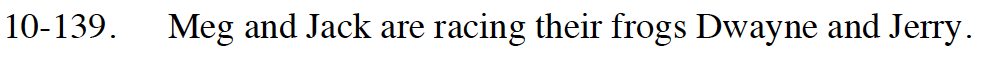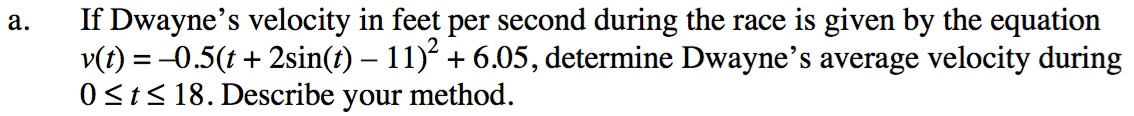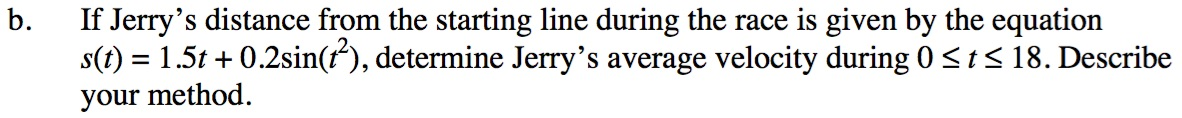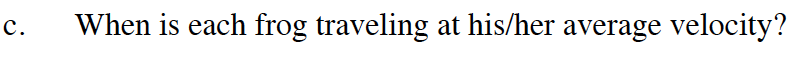### Home > CALC > Chapter 10 > Lesson 10.4.1 > Problem10-139

10-139.

Meg and Jack are racing their frogs Dwayne and Jerry.

1. If Dwayne’s velocity in feet per second during the race is given by the equation v(t) = –0.5(t + 2sin t – 11)2 + 6.05, determine Dwayne’s average velocity during 0 ≤ t ≤ 18. Describe your method.

2. If Jerry’s distance from the starting line during the race is given by the equation s(t) = 1.5x + 0.2sin x2, determine Jerry’s average velocity during 0 ≤ t ≤ 18. Describe your method.

3. When is each frog traveling at his/her average velocity?$v_{\text{avg}}=\frac{1}{18-0}\int_0^{18}v(t)dt$

Use a calculator to evaluate this integral.In this case, average velocity is total distance divided by total time.

$v_{\text{avg}}=\frac{s(18)-s(0)}{18-0}$